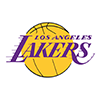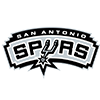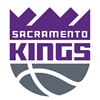DraftKings 2019 Western Conference Odds
+190
1.9 to 1Lakers
28.2% implied probability

+200
2 to 1Clippers
27.3% implied probability

+450
4.5 to 1Rockets
14.9% implied probability

+800
8 to 1Nuggets
9.1% implied probability

+900
9 to 1Jazz
8.2% implied probability

+1500
15 to 1Mavericks
5.1% implied probability

+4000
40 to 1Trail Blazers
2.0% implied probability

+6600
66 to 1Timberwolves
1.2% implied probability

+6600
66 to 1Suns
1.2% implied probability

+6600
66 to 1Spurs
1.2% implied probability

+20000
200 to 1Pelicans
0.4% implied probability

+20000
200 to 1Thunder
0.4% implied probability

+20000
200 to 1Kings
0.4% implied probability

+40000
400 to 1Warriors
0.2% implied probability

+40000
400 to 1Grizzlies
0.2% implied probability